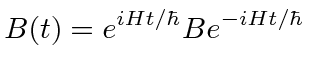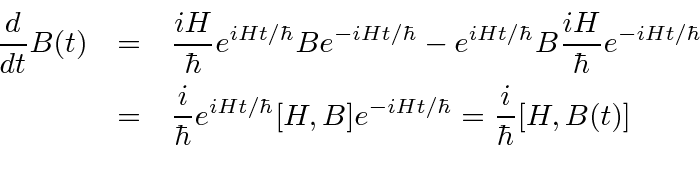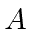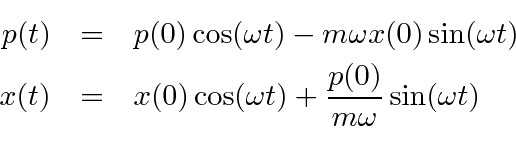## The Heisenberg Picture *

To begin, lets compute the expectation value of an operator.According to our rules, we can multiply operators together before using them. We can then define the operator that depends on time.If we use this operator, we don't need to do the time development of the wavefunctions!

This is called the Heisenberg Picture. In it, the operators evolve with time and the wavefunctions remain constant.

The usual Schrödinger picture has the states evolving and the operators constant.

We can now compute the time derivative of an operator.It is governed by the commutator with the Hamiltonian.

As an example, we may look at the HO operatorsand. We have already computed the commutator.We can integrate this.Take the Hermitian conjugate.We can combine these to get the momentum and position operators in the Heisenberg picture.Jim Branson 2013-04-22